﻿ Design and Analysis of Losses in Power TransformerPublications are Open
Access in this journal
Article Versions
Export Article
• Normal Style
• MLA Style
• APA Style
• Chicago Style
Original Article
Open Access Peer-reviewed

### Design and Analysis of Losses in Power Transformer

E.A. Anazia, E. S Ugochukwu , J. C. Onuegbu, Onyedikachi S.N
American Journal of Electrical and Electronic Engineering. 2017, 5(3), 94-101. DOI: 10.12691/ajeee-5-3-4
Published online: June 01, 2017

### Abstract

This paper examined and presented a simplified approach to power transformer design. Analyzed possible losses associated with power transformers through computational techniques and crucial design diagram.

### 1. Introduction

Transformer is a static electrical device that transfers energy by inductive coupling between its winding circuits 1. A varying current in the primary windings creates a varying magnetic flux through the secondary winding by which power is delivered to the load. Power transformer is slightly different from potential and current transformers in the sense that it transforms both current and voltage at the same time though they all work using the same principle of electromagnetic induction.

### 2. Construction Details of Transformer

Single phase shell type transformer is represented in the sketch below. Core type transformer exists, in which the primary and the secondary windings are located on the different limb. As the iron area of the leg Ai and the window area Aw = (Height of window L*Ww window width) increases, the size of the transformer also increases. In general the size of the transformer increases as the output of the transformer increases 2,

• Figure 1. Single phase shell type transformer

NB. W = Width of the transformer

H = Height of the transformer.

Ww = Ws = Window space width

Wc = Window space when insulation thickness is removed.

D = Thickness of the core

L = Height of the window

Lc = Window height when insulator is subtracted.

### 3. Winding Arrangement

The low voltage winding is placed next to the core and high voltage winding over the low voltage winding, on the central limb, in order to reduce the quantity of insulation used, avoid possibility of breakdown of space between the core and high voltage coil 3, 4, and to control the leakage reactance. However, in the case of low voltage rated transformers, low and high voltage coil can be arranged in any manner, as in this design, but to obtain optimum performance, we arranged the coils as shown below.

• Figure 2. Winding arrangement

### 4. Core Design

Net iron Area of the leg or limb or Core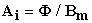(1)

The effect of mechanical forces on the wound coil under short circuit or high overload condition tends to deform the geometry of the transformer or its structure. These forces destroys the coil and insulations, in rectangular or square wound coil and core, whereas the effect is negligible in the case of circular wound coil and core or cruciform core design 5, 6. The circular core calls for more number of different sizes of laminations and poses a problem during core anchoring. Hence cruciform core design is preferred in practical design of transformers. Cruciform core design, reduces the net area of the core, gives high space factor, reduces the mean length of turns, and consequent I2R loss, as demonstrated in the two core design comparison below.

### 5. Leg or Limb Section Details

1. Square core (With a circular coil)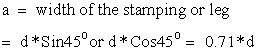(2)

where d is the diameter of the circumscribing circle.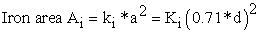(3)

= 0.9*0.5*d2 for 10% insulation or Ki = 0.9 (Iron or staking factor)

• Figure 3. Square cores with circular coil

Therefore Iron area;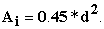(4)

Area of circumscribing circle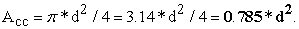(5)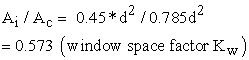(6)

NB: It is clear that Ai is only 57.3% of Ac. Rest of the area 42.7% of Ac is not being utilized usefully. In other to utilize the area usefully, more number of stepping of the core is necessary as shown below.

2. Cruciform or 2 stepped core and circular coil.

a = width of the largest stamping

b = width of the smallest stamping

Gross area of the core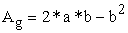(7)

Since a = d*Cosɵ, and b = d*Sinɵ -from the figure above.

Substituting in equation (7)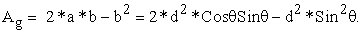(8)
• Figure 4. Cruciform cores with circular coil

By trigonometric identities,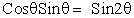(9)

Therefore,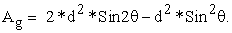(10)

For Ag = to be maximum, Ag must be differentiated with respect to ɵ, Ag = d2*(Sin2ɵ - Sin2 ɵ)

Hence;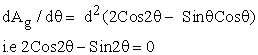(11)

= 2Cos2ɵ = Sin2ɵ.

2 = Sin2ɵ/Cos2ɵ = Tan2ɵ, Therefore,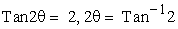(12)

ɵ = Tan-12/2 = 63.43/2 = 31.71o.

Thus, Ag is maximum when ɵ = 31.71o. With ɵ = 31.71o,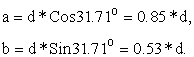(13)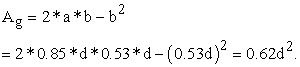(14)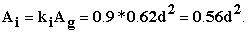(15)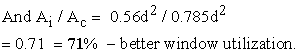(16)

### 6. Mean Length of Turns

For a given Area Ai say 10cm2,

(a) Square core (with a circular coil)

Ai = 0.45*d2 - as obtained in the previous analysis of equation (3).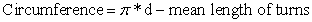(17)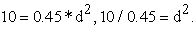Therefore: d = √22.22 = 4.71

Hence,(18)

(b) Cruciform-2 stepped core with circular coil.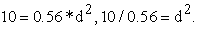Therefore: d = √17.857 = 4.226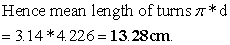(19)

Therefore, the addition of one step to the square core enhanced the utilization of more space of the circumscribing circle and reduced the mean length of turns by a difference of 1.52cm for the same number of turns. This result in more copper savings; i.e better economy of design.

### 7. Yoke Section Details.

The purpose of the yoke is to connect the legs providing a least reluctance path. In other to limit iron loss in the yoke, operating flux density is reduced by increasing yoke area. Generally, yoke area is made 20% more than the leg areas. i.e.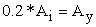(20)

Where Ay – yoke area

Ai – core or leg area.

### 8. Window Area and Core Proportion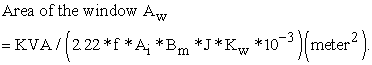(21)

If Hw = height of the window, Ww = width of the window, then Aw = Hw *Ww . In other to limit the leakage reactance of the transformer, Hw is made more than Ww. In practice, Hw/Ww lies between 2.5 and 3.5.

### 9. Winding Details

Since the applied voltage V1 is approximately equal to the voltage induced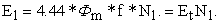(22)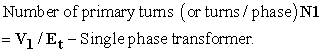(23)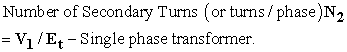(24)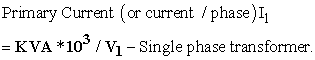(25)

Cross sectional Area of primary winding conductor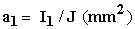(26)

Secondary current (or current/phase)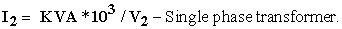(27)

Cross sectional area of secondary winding conductor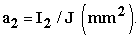(28)

### 9. Output Equations

The output equation for a Single phase shell type transformer is stated as follows: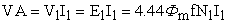(29)

Where Фm = Maximum flux, f = frequency of operation

N1 = Number of coil turns in the primary coil.

I1 = Electrical current in primary coil, which sets up the magnetizing flux.

4.44 – constant obtained from form factor.

### 10. Change of Flux in the Core

Since the flux is assumed to be sinusoidal, the maximum flux Фm is achieved at quarter the period of oscillation.

• Figure 5. Wave form of a sinusoidal flux

Hence, average rate of change of flux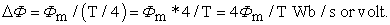(30)

But T = 1/f; this implies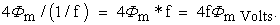(31)

Form factor = r.m.s value/average value = 1.11,

Therefore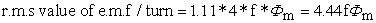(32)

Comparing with equation (29),

Power delivered VA = V1I1 = E1I1 = 4.44fФm N1 I1 or Power delivered per turn,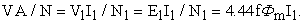(33)

But Фm = Bm*Ai , where Bm – maximum flux density, Ai = iron area or core area.

In a shell type transfer, there are two windows, which are symmetrical to each other. Hence it is sufficient to design one side. Since the low and high voltage windings are placed on the central limb, each window accommodates T1 and T2 turns of both primary and secondary windings.

Area of copper in the window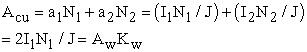(34)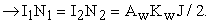(35)

Substituting in equation (29) above gives,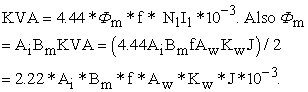(36)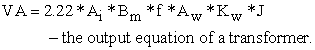(37)

### 11. Window Space factor

Window space factor is defined as the ratio of copper area in the window to the area of the window.

That is Kw = Area of copper in the window Acu / Area of the window Aw.

For a given window area, as the voltage rating of the transformer increases, quantity and quality of insulation in the window increases [7, 8] 7, 8, area of copper reduces. Thus the window space factor reduces as the voltage increases.

A value for Kw can be calculated by the following empirical formula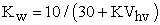(38)

Where KVhv or Vhv is the voltage of the high voltage winding expressed in KV or V.

### 12. Transformer Loss Analysis

Transformer losses fall into three categories:

1. No-load loss, or iron loss

2. Load-loss, copper loss, or short-circuit loss

3. Stray-loss (that is largely load related).

For some larger transformers there are also losses due to fans and pumps providing forced cooling. This will be discussed in a later example 9.

### 13. No Load Current of a Transformer

The no-load current I0 is the vectorial sum of the magnetizing current Im and core loss or working component current Ic. Function of Im is to produce flux Фm in the magnetic circuit and the function of Ic is to satisfy the no load losses of the transformer. Thus: I0 = √(Ic2 + Im2), Ampere.

• Figure 6. Vector diagram of transformer under no-load condition

No load input to the transformer = V1I0CosФ0 = V1Ic = No load losses as the output is zero and input = output + losses.

Since, the copper loss under no load condition is almost negligible, the no load losses can entirely be taken as due to core loss only. Thus the core loss component of the no load current Ic = Core loss/V1 for single phase transformer as in this case.

### 14. Transformer Approximation at no-load

1. In case of a transformer of normal design, the no load current will generally be less than about 2% of the full load current.

2. No load power factor Cos Ф0 = Ic/I0 and will be around 0.2.

3. Transformer copper loss.

(a) The primary copper loss, at no load is negligible as I0 is very less.

(b) The secondary copper loss is zero at no load, as no current flows in the secondary winding.

4. Core or Iron loss. Total core loss = loss in leg and yokes. The core loss can be estimated at design stage by referring to graph of core loss/kg versus flux density.

Core loss in leg = loss/kg in leg*weight of leg in kg = loss/kg in leg. = loss/kg in leg*volume of the leg (AiHw)*density of steel or iron used.

Core loss in yoke = loss/kg in yoke*volume of yoke (Ay*mean length of the yoke*density of iron used.)

NB: The density of iron or steel used for the transformer core design lies between 7.55-7.8 grams/cm2

The current I2 setup in the transformer when loaded is in phase with V2 if the load is resistive, lags behind V2 if the load is inductive, and leads it if the load is capacitive.

The presence of I2 in the secondary sets up demagnetizing ampere-turn Ф2, which reduces magnetizing ampere-turn Ф and V1 becomes higher than E1. The excesses of V1 causes more current I2 known as “load component of primary current” to flow, thereby setting up its own mmf Ф2. The interaction of this additional mmf cancels the effect of the demagnetizing mmf Ф2, previously set-up by I2 in the transformer.

Hence, whatever the load condition, the net flux passing through the core is approximately the same as at no-load. A vital deduction is that due to the constancy of core flux at all load; the “core loss” is also practically the same under all load conditions.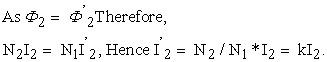Equivalent Resistance/Reluctance /Inductance of a Transformer

• Figure 7. Equivalent resistance of a transformer

The R2 – the equivalent secondary resistance as referred to primary. Copper loss in secondary is I22R2, which is supplied by primary which takes a current of I1.

Hence if R2 is the equivalent resistance in primary which would have caused the same loss as R2 in secondary, then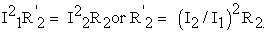(39)

Neglecting no-load current I0, then I2/I1 = 1/k, Where R2 = R2/K2. Referring R1 = to secondary, that is equivalent primary resistance referred to secondary, we have;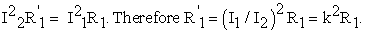(40)

### 16. Stray Loss

Winding Eddy Current Loss

Eddy-current loss is a significant additional component of winding loss. Winding eddy-currents are produced as a result of the alternating leakage flux cutting the windings. This flow within the conductor’s perpendicular to the load current path. The eddy-current losses are a fixed proportion of the load-losses for a particular winding. They do however vary as the square of the frequency, so that the presence of any harmonics in the load current leads to significant additional eddy-current loss 10.

For decades, eddy-current losses have presented an obstacle to reduction of I²R losses within transformer windings. This is because increasing the conductor cross-section with the objective of reducing winding resistance had the effect of worsening the eddy-current component, and little overall benefit was obtained. Since the mid-1960s, continuously transposed conductors which consist of a large number of individually enamelled insulated strands to increase the resistance of the eddy-current paths have been available and have largely eliminated this problem (Figure 8). Its use, coupled with flux shunts to control the distribution of leakage flux 11, means that eddy-current losses can now normally be contained within 10% to 15% of the I²R loss. Therefore, the reduction of load loss depends simply on the amount of materials copper and iron that is considered economical to put into the transformer.

• Figure 8. Eddy-currents in a solid conductor and in continuously transposed conductors.

Mechanical Metalwork Eddy Current Loss

These losses are those that occur in leads and tanks and other structural metalwork. Until the recent development of computer calculation techniques using finite element analysis, the magnitude of stray losses was usually determined empirically. The tolerances on guarantees took care of instances where designs did not quite conform to previous experience. Modern computer programmes have removed the uncertainty from this aspect of design and have facilitated improvements in the designs themselves 12. They enable designers to calculate and compare losses for differing arrangements as well as enabling suitable flux shields to be placed in critical areas. Stray loss, which is load dependent, has thus been reduced from perhaps 10% of the load losses to approximately half this value.

### 17. Sample Design

Specifications: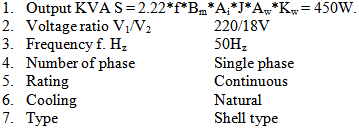Design Calculations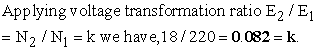(41)

Employing a two step cruciform core because of its gain,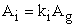(42)

Where ki = staking or iron factor

Ag = gross area of the core.

Assuming stacking factor of 0.9

Ai = 0.9*0.62d2, where d = diameter of the circumscribing circle or coil inner diameter or core diameter.

Assuming d = 5cm – by measurement.

Then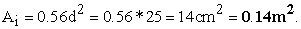(43)

Applying emf equation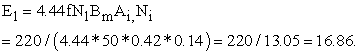(44)

18 turns – next even integer.

Using voltage transformation ratio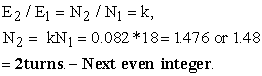(45)

Voltage per turn.- should be the same for both windings in an ideal design.

Therefore;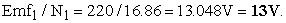(46)

Emf2/N2 = 18/1.48 = 12.162 = 12V » 1v difference accounts for stray loss (design loss)

Maximum flux ФmMaximum flux through the core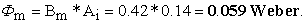(47)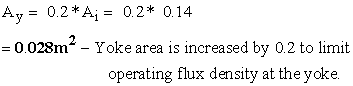(48)
• Figure 9. Single phase shell type transformer 

From the diagram above, overall length or width can be obtained as follows: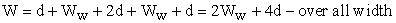(49)

H = L+2Ly where Ly = yoke height. Assuming Ly = d from the diagram, then;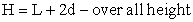(50)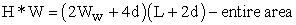(51)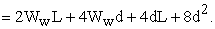(52)

Area of yoke and core = 0.168m2

Now, window area = 2WwL,

Therefore, Area of yoke and core = entire area (minus) window area.

That is;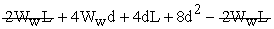–simplifying, we have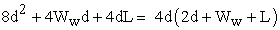(53)

NB: H/Ww = 2.5, therefore H = 2.5Ww = L, d = 5cm = 0.05m – as obtained from measurement as mentioned previously.

Substituting these values in the expression above we have,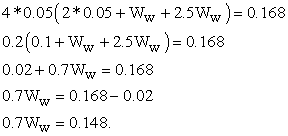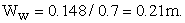(54)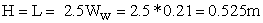(55)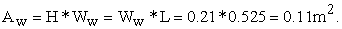(56)

Therefore,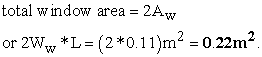(57)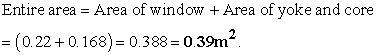(58)

Taking area covered by the insulator into consideration, and assuming the coil did not torch the two outer limbs, and then we have,

Insulation thickness = 2mm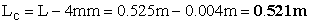(59)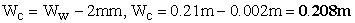(60)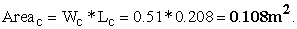(61)

Total window area when insulation thickness is subtracted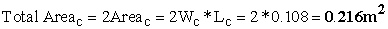(62)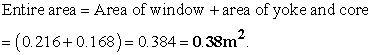(63)

Area of copper in the window or coil used Acu is obtained as follows;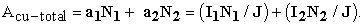(64)

From calculation we already know the following,

N1 = 18 turns, N2 = 2 turns, J = 1064 Ampere m-2, I1 = 2.56A, I2 = 31.25A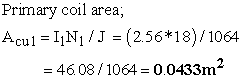(65)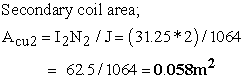(66)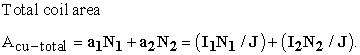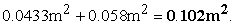(67)

Diameter of the coil used in the primary winding is obtained as follows

Acu1 = πd2/4; » 0.0433 = 3.142d2/4; » 0.1732 = 3.142d2;

Therefore;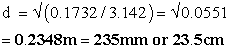(68)

i.e 0.2m – approximately.

Diameter of the coil used in the secondary winding is obtained as follows

Acu2 = πd2/4; » 0.058 = 3.142d2/4; » 0.2348 = 3.142d2

Therefore;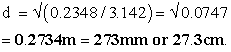(69)

i.e 0.3m – approximately.

Total window area by calculation result obtained previously taking insulation thickness into consideration;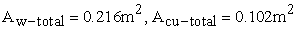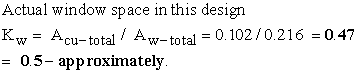(70)

Mean length of coil used in this design is calculated as follows;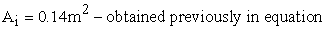(43)

Circumference of the core is approximated to πd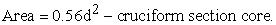Therefore; Ai = πd; » 0.14 = 0.56d2; » d2 = 0.14/0.56; » d = √0.25, = 0.5m

Therefore; mean length of turns,

For; secondary side,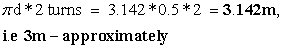(71)

For; primary side,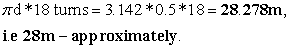(72)

Current drawn from the mains by the primary winding is obtained from,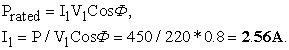(73)

Current to be delivered to load at the secondary terminal is obtained from,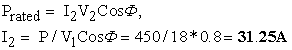(74)

Therefore power rating of the transformer is obtained as follows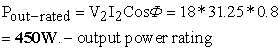(75)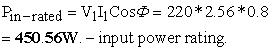(76)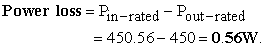(77)

Rated Power

Applying equation …..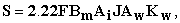(78)

we have F = 50Hz, Bm = 0.42 tesla, Ai = 0.14m2, Фm = 0.059 Weber

Aw = 0.216m2 (insulation thickness subtracted)

Kw = 0.3 – Window space factor

J = ?, S = Prated = 450W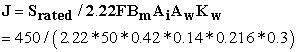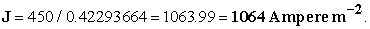(79)

Therefore Prated = Srated = 2.22FBmAi JAwKw

Prated = Srated = 2.22*50*0.42*0.14*1064*0.216*0.3 = 450.00458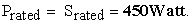(80)

Efficiency = power output/power input *100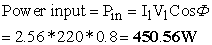(81)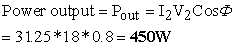(82)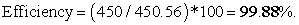(83)

### 18. Conclusion

This manuscript presented design principles of power transformer (VA-transformer), laying bare the basic design calculations required to design a functional transformer. The unique nature of this paper is seen in its simplicity and straight forwardness. It broke down transformer design process to easily understandable form and removed ambiguity usually introduced by complex computational techniques found in most design paper. Readers with average knowledge as well as professionals would find this paper a handy guide.

### References

  Design of Transformers, Retrieved from http://elearning.vtu.ac.in/16/ENotes/Elec%20Mac%20Des/Unit3-Era.pdf. In article View Article  GUIDE for Life Management Techniques for Power Transformers,” (CIGRE brochure No. 227). In article  L. Pettersson, N.L. Fantana, U. Sunderman. “Assessment ranking of Power Transformers Using Condition Based Evaluation, A New Approach”, CIGRE Paris Conference Paper 12-204, 1998 Session. In article  Mark Perkins, Lars Pettersson, Nicolai Fantana, T. V. Oommen, and Steven Jordan. “Transformer life assessment tools and methods” Proceedings of the 2000 International Conference of Doble Clients - Sec 8-1. In article  Stefan Fassbinder (2013). Efficiency and Loss Evaluation of Large Power Tranformers, ECI Publication No Cu0144, Issue 01, Available from www.leonardo-energy.org. In article  Transformer, Retrieved from http://en.wikipedia.org/wiki/Transformer. In article View Article  Victor Sokolov, Armando Bassetto, T.V. Oommen, Ted Haupert, and Dave Hanson. ”Transformer fluid:a powerful tool for the life management of an ageing transformer population” Proceedings of the TechCon 2003 Asia-Pacific. In article View Article  Victor Sokolov, Armando Bassetto, Jose Mak, Dave Hanson. “Transformer Risk Assessment Considerations’ Proceedings of the EuroTechCon 2002 Conference,UK Bimingham, 2002. In article  V.Sokolov. “Consideration on power transformer condition –based maintenance”, Proceeding of the EPRI Substation Equipment Diagnostic Conference VIII. February 20-23, 2000, New Orleans, LA. In article View Article  V. Sokolov. “Changing world perspectives-a report from CIGRE”, Proceedings of the TechCon N.A. 2005 conference,San Diego, Feb.2005. In article  V. Sokolov. “Design Review as the First Step of Transformer Life Assessment”, Proceedings of the EuroTechCon Conference, Birmingham, 2003. In article  Balbir Sngh (1982). Electrical Machine Design; Vikas Publishing House PVT ltd. In articleThis work is licensed under a Creative Commons Attribution 4.0 International License. To view a copy of this license, visit http://creativecommons.org/licenses/by/4.0/